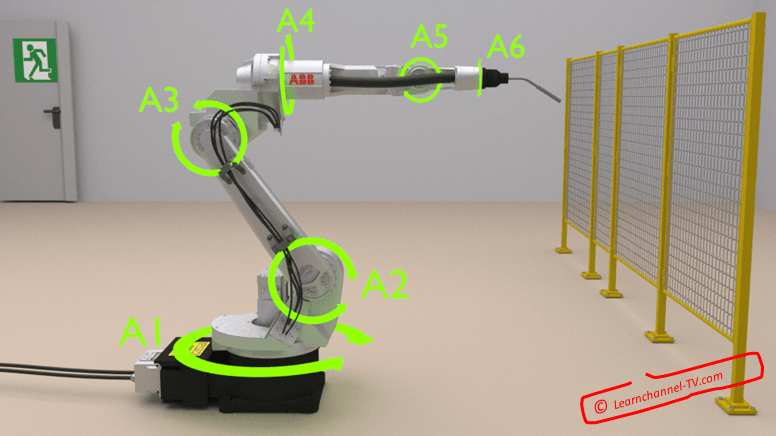# Joint coordinates

## Robot JOINT-coordinates

The angle-position and length of each axis of an articulate robot axes describe the orientation of the TCP exactly. With the joint coordinate-system each robot axis can be moved particularly in positive or negative sense rotation.

Coordinates:    P (angle A1, angle A2, ... , angle A6)fig.:    Robot joint coordinates - Learnchannel